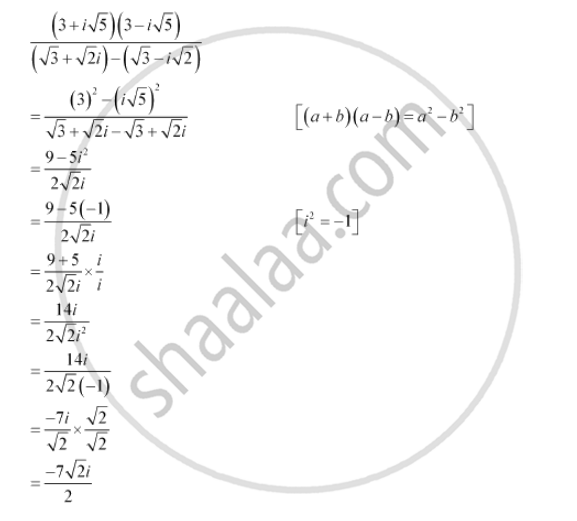CBSE (Arts) Class 11CBSE
Share

# Express the Following Expression in the Form Of A + Ib. ((3 + Sqrt5)(3 - Isqrt5))/((Sqrt3 + Sqrt2i)-(Sqrt3 - Isqrt2)) - CBSE (Arts) Class 11 - Mathematics

ConceptAlgebra of Complex Numbers

#### Question

Express the following expression in the form of a + ib.

((3 + sqrt5)(3 - isqrt5))/((sqrt3 + sqrt2i)-(sqrt3 - isqrt2))

#### SolutionIs there an error in this question or solution?

#### Video TutorialsVIEW ALL 

Solution Express the Following Expression in the Form Of A + Ib. ((3 + Sqrt5)(3 - Isqrt5))/((Sqrt3 + Sqrt2i)-(Sqrt3 - Isqrt2)) Concept: Algebra of Complex Numbers.
S